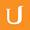## SD of Standardized Distribution - Intro to Descriptive Statistics

• 0:00 - 0:04
Now remember, when we calculate the z score of any value in the distribution,
• 0:04 - 0:09
we first subtract the mean which shifts the distribution without changing the
• 0:09 - 0:15
shape so that zero is now the mean. And when we divide by the standard
• 0:15 - 0:21
deviation, we then change the shape. Let's look at it this way. We have any
• 0:21 - 0:27
distribution, with mean, mu, and standard deviation, sigma. Which basically
• 0:27 - 0:31
means that sigma is one standard deviation away from the mean. After we
• 0:31 - 0:36
standardize this distribution, what is going to be the z-score of sigma? Well,
• 0:36 - 0:43
remember when we subtract mu, we shift it so that mu is now zero. So now, the
• 0:43 - 0:48
z-score of sigma is going to be sigma minus zero divided by sigma, which is
• 0:48 - 0:55
sigma divided by sigma, which is 1. So, the z-score of any value, that's one
• 0:55 - 1:03
standard deviation away from the mean, will then be 1 after we standardize it.
• 1:03 - 1:07
Which means that the new standard deviation of this normalized distribution, or
• 1:07 - 1:10
standard distribution, is 1.
Tytuł:
SD of Standardized Distribution - Intro to Descriptive Statistics
Opis:

more » « less
Video Language:
English
Team:Udacity
Projekt:
UD827 - Intro to Descriptive Statistics
Duration:
01:11Udacity Robot edited angielski subtitles for 10-34 SD of Standardized DistributionUdacity Robot edited angielski subtitles for 10-34 SD of Standardized DistributionCogi-Admin added a translation

# English subtitles

## Revisions Compare revisions

• API
Udacity Robot
• API
Udacity Robot